# 基于事件触发控制带有参数不确定的时滞Lur’e系统主从同步Master-Slave Synchronization of Time-Delay Lur’e Systems with Parametric Uncertainty Based on the Event-Triggered Mechanism

DOI: 10.12677/AAM.2021.104140, PDF, HTML, XML, 下载: 12  浏览: 30  科研立项经费支持

Abstract: This paper investigates the master-slave synchronization of sampled-data for delay Lur’e systems with uncertain parameters. In order to save the channel resources, the aperiodic event-triggered mechanism is introduced. Only when the trigger condition is satisfied, the current sampled-data will be transferred to the network. In order to reduce the conservatism and make full use of the nonlinearity of the system, the appropriate triple Lyapunov-Krasovskii functional terms are introduced. It is not required that all symmetric matrices are positive definite matrices. A new synchronization criterion in the form of linear matrix inequalities (LMIs) is given by combining the integral inequality based on auxiliary function, inverse convex matrix inequality and Schur complement lemma. Finally, two numerical simulations of Chua’s circuit are provided to show the effectiveness of the conclusion.

1. 引言

2. 问题描述

$M:\left\{\begin{array}{c}\stackrel{˙}{x}\left(t\right)=\left(A+\Delta Α\left(t\right)\right)x\left(t\right)+\left(B+\Delta B\left(t\right)\right)x\left(t-\tau \left(t\right)\right)+\left(E+\Delta E\left(t\right)\right)f\left(Dx\left(t\right)\right)\\ p\left(t\right)=Cx\left(t\right)\text{\hspace{0.17em}}\text{\hspace{0.17em}}\text{\hspace{0.17em}}\text{\hspace{0.17em}}\text{\hspace{0.17em}}\text{\hspace{0.17em}}\text{\hspace{0.17em}}\text{\hspace{0.17em}}\text{\hspace{0.17em}}\text{\hspace{0.17em}}\text{\hspace{0.17em}}\text{\hspace{0.17em}}\text{\hspace{0.17em}}\text{\hspace{0.17em}}\text{\hspace{0.17em}}\text{\hspace{0.17em}}\text{\hspace{0.17em}}\text{\hspace{0.17em}}\text{\hspace{0.17em}}\text{\hspace{0.17em}}\text{\hspace{0.17em}}\text{\hspace{0.17em}}\text{\hspace{0.17em}}\text{\hspace{0.17em}}\text{\hspace{0.17em}}\text{\hspace{0.17em}}\text{\hspace{0.17em}}\text{\hspace{0.17em}}\text{\hspace{0.17em}}\text{\hspace{0.17em}}\text{\hspace{0.17em}}\text{\hspace{0.17em}}\text{\hspace{0.17em}}\text{\hspace{0.17em}}\text{\hspace{0.17em}}\text{\hspace{0.17em}}\text{\hspace{0.17em}}\text{\hspace{0.17em}}\text{\hspace{0.17em}}\text{\hspace{0.17em}}\text{\hspace{0.17em}}\end{array}$ (1)

$S\text{\hspace{0.17em}}\text{\hspace{0.17em}}:\left\{\begin{array}{c}\stackrel{˙}{y}\left(t\right)=\left(A+\Delta Α\left(t\right)\right)y\left(t\right)+\left(B+\Delta B\left(t\right)\right)y\left(t-\tau \left(t\right)\right)+\left(E+\Delta E\left(t\right)\right)f\left(Dy\left(t\right)\right)+U\left(t\right)\\ q\left(t\right)=Cy\left(t\right)\text{\hspace{0.17em}}\text{\hspace{0.17em}}\text{\hspace{0.17em}}\text{\hspace{0.17em}}\text{\hspace{0.17em}}\text{\hspace{0.17em}}\text{\hspace{0.17em}}\text{\hspace{0.17em}}\text{\hspace{0.17em}}\text{\hspace{0.17em}}\text{\hspace{0.17em}}\text{\hspace{0.17em}}\text{\hspace{0.17em}}\text{\hspace{0.17em}}\text{\hspace{0.17em}}\text{\hspace{0.17em}}\text{\hspace{0.17em}}\text{\hspace{0.17em}}\text{\hspace{0.17em}}\text{\hspace{0.17em}}\text{\hspace{0.17em}}\text{\hspace{0.17em}}\text{\hspace{0.17em}}\text{\hspace{0.17em}}\text{\hspace{0.17em}}\text{\hspace{0.17em}}\text{\hspace{0.17em}}\text{\hspace{0.17em}}\text{\hspace{0.17em}}\text{\hspace{0.17em}}\text{\hspace{0.17em}}\text{\hspace{0.17em}}\text{\hspace{0.17em}}\text{\hspace{0.17em}}\text{\hspace{0.17em}}\text{\hspace{0.17em}}\text{\hspace{0.17em}}\text{\hspace{0.17em}}\text{\hspace{0.17em}}\text{\hspace{0.17em}}\text{\hspace{0.17em}}\text{\hspace{0.17em}}\text{\hspace{0.17em}}\text{\hspace{0.17em}}\text{\hspace{0.17em}}\text{\hspace{0.17em}}\text{\hspace{0.17em}}\text{\hspace{0.17em}}\text{\hspace{0.17em}}\text{\hspace{0.17em}}\end{array}$ (2)

$0\le {\tau }_{1}\le \tau \left(t\right)\le {\tau }_{2},{u}_{1}\le \stackrel{˙}{\tau }\left(t\right)\le {u}_{2}$ (3)

${k}_{i}^{-}\le \frac{{f}_{i}\left({s}_{1}\right)-{f}_{i}\left({s}_{2}\right)}{{s}_{1}-{s}_{2}}\le {k}_{i}^{+},\text{\hspace{0.17em}}\text{\hspace{0.17em}}\text{\hspace{0.17em}}{s}_{1}\ne {s}_{2},\text{}i=1,2,\cdots ,m$ (4)

$\left[\Delta A\left(t\right),\Delta B\left(t\right),\Delta E\left(t\right)\right]=M\Delta \left(t\right)\left[{N}_{1},{N}_{2},{N}_{3}\right]$ (5)

${\Delta }^{\text{T}}\left(t\right)\Delta \left(t\right)\le I$ (6)

$0\le r\left(t\right)=t-{t}_{s}\le h,\text{\hspace{0.17em}}\text{\hspace{0.17em}}\text{\hspace{0.17em}}\forall s\ge 0$ (7)

$C:U\left(t\right)=K\left(p\left({t}_{k}\right)-q\left({t}_{k}\right)\right),\text{\hspace{0.17em}}\text{\hspace{0.17em}}\text{\hspace{0.17em}}{t}_{k}\le t<{t}_{k+1}$ (8)

$e\left(t\right)=x\left(t\right)-y\left(t\right)$，由(1)、(2)、(8)可以获得同步误差系统：

$\begin{array}{l}\stackrel{˙}{e}\left(t\right)=\left(A+\Delta A\left(t\right)\right)e\left(t\right)+\left(B+\Delta B\left(t\right)\right)e\left(t-\tau \left(t\right)\right)\\ \text{\hspace{0.17em}}\text{\hspace{0.17em}}\text{\hspace{0.17em}}\text{\hspace{0.17em}}\text{\hspace{0.17em}}\text{\hspace{0.17em}}\text{\hspace{0.17em}}\text{\hspace{0.17em}}+\left(E+\Delta E\left(t\right)\right)g\left(De\left(t\right)\right)-KCe\left({t}_{k}\right),\text{\hspace{0.17em}}\text{\hspace{0.17em}}\text{\hspace{0.17em}}{t}_{k}\le t<{t}_{k+1}\end{array}$ (9)

${k}_{i}^{-}\le \frac{{g}_{i}\left({d}_{i}^{\text{T}}e,y\right)}{{d}_{i}^{\text{T}}e}=\frac{{f}_{i}\left({d}_{i}^{\text{T}}\left(y+e\right)\right)-{f}_{i}\left({d}_{i}^{\text{T}}y\right)}{{d}_{i}^{\text{T}}e}\le {k}_{i}^{+},\text{\hspace{0.17em}}\text{\hspace{0.17em}}\text{\hspace{0.17em}}{d}_{i}^{\text{T}}e\ne 0$ (10)

${\epsilon }^{\text{T}}\left({t}_{s}\right){\Theta }_{1}\epsilon \left({t}_{s}\right)-{\sigma }_{1}\left(t\right){e}^{\text{T}}\left({t}_{s}\right){\Theta }_{2}e\left({t}_{s}\right)-{\sigma }_{2}\left(t\right){e}^{\text{T}}\left({t}_{k}\right){\Theta }_{3}e\left({t}_{k}\right)\le 0$ (11)

${\stackrel{˙}{\sigma }}_{1}\left(t\right)=\frac{1}{{\sigma }_{1}\left(t\right)}\left[\frac{1}{{\sigma }_{1}\left(t\right)}-{\mu }_{1}\right]\left[{\epsilon }^{\text{T}}\left({t}_{s}\right){\Theta }_{1}\epsilon \left({t}_{s}\right)-{\kappa }_{1}{e}^{\text{T}}\left({t}_{k}\right){\Theta }_{3}e\left({t}_{k}\right)\right]\text{\hspace{0.17em}}$ (12)

${\stackrel{˙}{\sigma }}_{2}\left(t\right)=\frac{1}{{\sigma }_{2}\left(t\right)}\left[\frac{1}{{\sigma }_{2}\left(t\right)}-{\mu }_{2}\right]\left[{\epsilon }^{\text{T}}\left({t}_{s}\right){\Theta }_{1}\epsilon \left({t}_{s}\right)-{\kappa }_{2}{e}^{\text{T}}\left({t}_{s}\right){\Theta }_{2}e\left({t}_{s}\right)\right]$ (13)

$\begin{array}{l}\stackrel{˙}{e}\left(t\right)=\left(A+\Delta A\left(t\right)\right)e\left(t\right)+\left(B+\Delta B\left(t\right)\right)e\left(t-\tau \left(t\right)\right)\\ \text{\hspace{0.17em}}\text{\hspace{0.17em}}\text{\hspace{0.17em}}\text{\hspace{0.17em}}\text{\hspace{0.17em}}\text{\hspace{0.17em}}\text{\hspace{0.17em}}\text{\hspace{0.17em}}+\left(E+\Delta E\left(t\right)\right)g\left(De\left(t\right)\right)-KCe\left({t}_{s}\right)+K\epsilon \left({t}_{s}\right),\text{\hspace{0.17em}}\text{\hspace{0.17em}}\text{\hspace{0.17em}}t\in {H}_{s}\end{array}$ (14)

$\left(b-a\right){\int }_{a}^{b}{\omega }^{T}\left(s\right)\text{\hspace{0.17em}}R\omega \left(s\right)\text{d}s\ge {\left({\int }_{a}^{b}\omega \left(s\right)\text{\hspace{0.17em}}\text{d}s\right)}^{\text{T}}R\left({\int }_{a}^{b}\omega \left(s\right)\text{\hspace{0.17em}}\text{d}s\right)+3{\theta }^{\text{T}}R\theta$

$\begin{array}{l}{\int }_{a}^{b}{\stackrel{˙}{x}}^{T}\left(s\right)R\stackrel{˙}{x}\left(s\right)\text{d}s\ge \frac{1}{b-a}{\Omega }_{1}^{T}R{\Omega }_{1}+\frac{3}{b-a}{\Omega }_{2}^{T}R{\Omega }_{2}+\frac{5}{b-a}{\Omega }_{3}^{T}R{\Omega }_{3}\\ {\int }_{a}^{b}{\int }_{\theta }^{b}{\stackrel{˙}{x}}^{T}\left(s\right)\text{\hspace{0.17em}}R\stackrel{˙}{x}\left(s\right)\text{d}s\text{d}\theta \ge 2{\Omega }_{4}^{T}R{\Omega }_{4}+4{\Omega }_{5}^{T}R{\Omega }_{5}\end{array}$

$\begin{array}{l}{\Omega }_{1}=x\left(b\right)-x\left(a\right),\text{}{\Omega }_{2}=x\left(b\right)+x\left(a\right)-\frac{1}{b-a}{\int }_{a}^{b}x\left(s\right)\text{\hspace{0.17em}}\text{d}s\\ {\Omega }_{3}={\Omega }_{1}+\frac{6}{b-a}{\int }_{a}^{b}x\left(s\right)\text{\hspace{0.17em}}\text{d}s-\frac{12}{{\left(b-a\right)}^{2}}{\int }_{a}^{b}{\int }_{\theta }^{b}x\left(s\right)\text{d}s\text{d}\theta \\ {\Omega }_{4}=x\left(b\right)-\frac{1}{b-a}{\int }_{a}^{b}x\left(s\right)\text{\hspace{0.17em}}\text{d}s,\text{}{\Omega }_{5}=x\left(b\right)+\frac{2}{b-a}{\int }_{a}^{b}x\left(s\right)\text{\hspace{0.17em}}\text{d}s-\frac{6}{{\left(b-a\right)}^{2}}{\int }_{a}^{b}{\int }_{\theta }^{b}x\left(s\right)\text{d}s\text{d}\theta \end{array}$

$\left(\begin{array}{cc}\frac{1}{\alpha }{R}_{1}& \\ & \frac{1}{\beta }{R}_{2}\end{array}\right)\ge \left(\begin{array}{cc}{R}_{1}+\left(1-\alpha \right){P}_{1}& \left(1-\alpha \right){S}_{1}+\alpha {S}_{2}\\ \ast & {R}_{2}+\alpha {P}_{2}\end{array}\right)$

$\Psi +{\epsilon }^{-1}\Gamma {\Gamma }^{\text{T}}+\epsilon {\Xi }^{\text{T}}\Xi <0$

$\begin{array}{l}\text{1)}S<0;\\ 2\right)\text{}{S}_{11}<0,{S}_{22}-{S}_{12}^{T}{S}_{11}^{-1}{S}_{12}<0;\\ 3\right)\text{}{S}_{22}<0,{S}_{11}-{S}_{12}{S}_{22}^{-1}{S}_{12}^{T}<0;\end{array}$

$\Omega +\left(\tau \left(t\right)-{\tau }_{m}\right){\Xi }_{1}+\left({\tau }_{M}-\tau \left(t\right)\right){\Xi }_{2}+h\left(t\right){\Xi }_{3}+\left({h}_{M}-h\left(t\right)\right){\Xi }_{4}<0$

$\begin{array}{l}\Omega +\left({\tau }_{M}-{\tau }_{m}\right){\Xi }_{1}+{h}_{M}{\Xi }_{3}<0\\ \Omega +\left({\tau }_{M}-{\tau }_{m}\right){\Xi }_{2}+{h}_{M}{\Xi }_{3}<0\\ \Omega +\left({\tau }_{M}-{\tau }_{m}\right){\Xi }_{1}+{h}_{M}{\Xi }_{4}<0\\ \Omega +\left({\tau }_{M}-{\tau }_{m}\right){\Xi }_{2}+{h}_{M}{\Xi }_{4}<0\end{array}$

$\begin{array}{l}\xi \left(t\right)=col\left\{e\left(t\right),e\left(t-{\tau }_{1}\right),e\left(t-\tau \left(t\right)\right),e\left(t-{\tau }_{2}\right),e\left({t}_{s}\right),g\left(De\left(t\right)\right),\frac{1}{{\tau }_{1}}{\int }_{t-{\tau }_{1}}^{t}e\left(s\right)\text{\hspace{0.17em}}\text{d}s,\frac{1}{\tau \left(t\right)-{\tau }_{1}}{\int }_{t-\tau \left(t\right)}^{t-{\tau }_{1}}e\left(s\right)\text{\hspace{0.17em}}\text{d}s,\\ \text{\hspace{0.17em}}\text{\hspace{0.17em}}\text{\hspace{0.17em}}\text{\hspace{0.17em}}\text{\hspace{0.17em}}\text{\hspace{0.17em}}\text{\hspace{0.17em}}\text{\hspace{0.17em}}\text{\hspace{0.17em}}\frac{1}{{\tau }_{2}-\tau \left(t\right)}{\int }_{t-{\tau }_{2}}^{t-\tau \left(t\right)}e\left(s\right)\text{\hspace{0.17em}}\text{d}s,\frac{1}{t-{t}_{s}}{\int }_{{t}_{s}}^{t}e\left(s\right)\text{\hspace{0.17em}}\text{d}s,\frac{2}{{\tau }_{1}^{2}}{\int }_{t-{\tau }_{1}}^{t}{\int }_{\theta }^{t}e\left(s\right)\text{\hspace{0.17em}}\text{d}s\text{d}\theta ,\frac{2}{{\left[\tau \left(t\right)-{\tau }_{1}\right]}^{2}}{\int }_{t-\tau \left(t\right)}^{t-{\tau }_{1}}{\int }_{\theta }^{t-{\tau }_{1}}e\left(s\right)\text{\hspace{0.17em}}\text{d}s\text{d}\theta ,\\ \text{\hspace{0.17em}}\text{\hspace{0.17em}}\text{\hspace{0.17em}}\text{\hspace{0.17em}}\text{\hspace{0.17em}}\text{\hspace{0.17em}}\text{\hspace{0.17em}}\text{\hspace{0.17em}}\text{\hspace{0.17em}}\frac{2}{{\left[{\tau }_{2}-\tau \left(t\right)\right]}^{2}}{\int }_{t-{\tau }_{2}}^{t-\tau \left(t\right)}{\int }_{\theta }^{t-\tau \left(t\right)}e\left(s\right)\text{\hspace{0.17em}}\text{d}s\text{d}\theta ,\frac{1}{{\left(t-{t}_{s}\right)}^{2}}{\int }_{{t}_{s}}^{t}{\int }_{\theta }^{t}e\left(s\right)\text{\hspace{0.17em}}\text{d}s\text{d}\theta ,\stackrel{˙}{e}\left(t\right),\epsilon \left({t}_{s}\right)\right\}\end{array}$

${\eta }_{1}\left(t\right)=col\left\{e\left(t\right),\text{\hspace{0.17em}}\text{\hspace{0.17em}}e\left({t}_{s}\right),\text{\hspace{0.17em}}\text{\hspace{0.17em}}{\int }_{{t}_{s}}^{t}e\left(s\right)\mathrm{d}s\right\}$

$X=\left(\begin{array}{ccc}\frac{{X}_{1}^{\text{T}}+{X}_{1}-{X}_{2}^{\text{T}}-{X}_{2}}{2}& {X}_{2}& {X}_{3}\\ \ast & \frac{-{X}_{1}^{\text{T}}-{X}_{1}-{X}_{2}^{\text{T}}-{X}_{2}}{2}& {X}_{4}\\ \ast & \ast & {X}_{5}^{\text{T}}+{X}_{5}\end{array}\right)$

$\begin{array}{l}L=col\left\{{G}^{\text{T}}\text{\hspace{0.17em}}\text{\hspace{0.17em}}0\text{\hspace{0.17em}}\text{\hspace{0.17em}}0\text{\hspace{0.17em}}\text{\hspace{0.17em}}0\text{\hspace{0.17em}}\text{\hspace{0.17em}}{G}^{\text{T}}\text{\hspace{0.17em}}\text{\hspace{0.17em}}0\text{\hspace{0.17em}}\text{\hspace{0.17em}}0\text{\hspace{0.17em}}\text{\hspace{0.17em}}0\text{\hspace{0.17em}}\text{\hspace{0.17em}}0\text{\hspace{0.17em}}\text{\hspace{0.17em}}0\text{\hspace{0.17em}}\text{\hspace{0.17em}}0\text{\hspace{0.17em}}\text{\hspace{0.17em}}0\text{\hspace{0.17em}}\text{\hspace{0.17em}}0\text{\hspace{0.17em}}\text{\hspace{0.17em}}0\text{\hspace{0.17em}}\text{\hspace{0.17em}}{G}^{\text{T}}\text{\hspace{0.17em}}\text{\hspace{0.17em}}0\right\}\\ N=\left\{{N}_{1}\text{\hspace{0.17em}}\text{\hspace{0.17em}}0\text{\hspace{0.17em}}\text{\hspace{0.17em}}{N}_{2}\text{\hspace{0.17em}}\text{\hspace{0.17em}}0\text{\hspace{0.17em}}\text{\hspace{0.17em}}0\text{\hspace{0.17em}}\text{\hspace{0.17em}}{N}_{3}\text{\hspace{0.17em}}\text{\hspace{0.17em}}0\text{\hspace{0.17em}}\text{\hspace{0.17em}}0\text{\hspace{0.17em}}\text{\hspace{0.17em}}0\text{\hspace{0.17em}}\text{\hspace{0.17em}}0\text{\hspace{0.17em}}\text{\hspace{0.17em}}0\text{\hspace{0.17em}}\text{\hspace{0.17em}}0\text{\hspace{0.17em}}\text{\hspace{0.17em}}0\text{\hspace{0.17em}}\text{\hspace{0.17em}}0\text{\hspace{0.17em}}\text{\hspace{0.17em}}0\text{\hspace{0.17em}}\text{\hspace{0.17em}}0\right\}\end{array}$

${e}_{i}=\left(\begin{array}{ccc}{0}_{n×\left(i-1\right)n}& {I}_{n}& {0}_{n×\left[\left(15-i\right)n+m\right]}\end{array}\right),i=1,2,\cdots ,5\text{ }\text{ }\text{ }\text{ }\text{ }\text{ }\text{ }\text{ }\text{ }\text{ }\text{ }\text{ }{e}_{6}=\left(\begin{array}{ccc}{0}_{m×5n}& {I}_{m}& {0}_{m×10n}\end{array}\right)$

${e}_{i}=\left(\begin{array}{ccc}{0}_{n×\left[\left(i-2\right)n+m\right]}& {I}_{n}& {0}_{n×\left(16-i\right)n}\end{array}\right),\text{}i=7,8,\cdots ,16$

${E}_{1}=\left(\begin{array}{c}{e}_{2}-{e}_{3}\\ {e}_{2}+{e}_{3}-2{e}_{8}\\ {e}_{2}-{e}_{3}+6{e}_{8}-6{e}_{12}\end{array}\right),{E}_{2}=\left(\begin{array}{c}{e}_{3}-{e}_{4}\\ {e}_{3}+{e}_{4}-2{e}_{9}\\ {e}_{3}-{e}_{4}+6{e}_{9}-6{e}_{13}\end{array}\right)$

3. 主要结果

$\left(\begin{array}{ccc}P+\frac{h\left({X}_{1}^{\text{T}}+{X}_{1}-{X}_{2}^{\text{T}}-{X}_{2}\right)}{2}& h{X}_{2}& h{X}_{3}\\ \ast & \frac{-h\left({X}_{1}^{\text{T}}+{X}_{1}+{X}_{2}^{\text{T}}+{X}_{2}\right)}{2}& h{X}_{4}\\ \ast & \ast & h\left({X}_{5}^{\text{T}}+{X}_{5}\right)\end{array}\right)>0$ (15)

$\left(\begin{array}{cccc}{\Psi }_{1}+{\Psi }_{i}+{\Psi }_{4,1}& {P}_{2}& LM& \epsilon {N}^{\text{T}}\\ \ast & {\stackrel{¯}{J}}_{4}& 0& 0\\ \ast & \ast & -\epsilon I& 0\\ \ast & \ast & \ast & -\epsilon I\end{array}\right)<0$ (16)

$\left(\begin{array}{cccc}{\Psi }_{1}+{\Psi }_{i}+{\Psi }_{4,2}& {P}_{1}^{\text{T}}& LM& \epsilon {N}^{\text{T}}\\ \ast & {\stackrel{¯}{J}}_{4}+{\stackrel{¯}{J}}_{6}& 0& 0\\ \ast & \ast & -\epsilon I& 0\\ \ast & \ast & \ast & -\epsilon I\end{array}\right)<0$ (17)

0元素除外， ${\Psi }_{1}={\left[{\Psi }_{ij}\right]}_{16×16}$ 的剩余元素如下：

${\Psi }_{11}=Sym\left\{\left({X}_{2}-{X}_{1}\right)/2-{D}^{\text{T}}{K}_{2}^{\text{T}}F{K}_{1}D+GA\right\}-6{R}_{3}-\left({\text{π}}^{2}/4\right)Q+{J}_{1}-9{J}_{3}-6{J}_{5},{\Psi }_{12}=3{J}_{3},{\Psi }_{13}=GB,$

${\Psi }_{15}=-{X}_{2}+\frac{{\text{π}}^{2}}{4}Q+{A}^{\text{T}}{G}^{\text{T}}-YC,{\Psi }_{16}=GE+{D}^{\text{T}}{K}_{1}^{\text{T}}{F}^{\text{T}}+{D}^{\text{T}}{K}_{2}^{\text{T}}F,{\Psi }_{17}=-24{J}_{3}-6{J}_{5},$

${\Psi }_{1,10}=\left({h}^{2}/4\right)Sym\left\{{X}_{5}\right\}-{R}_{22}-6{R}_{3},{\Psi }_{1,11}=30{J}_{3}+12{J}_{5},{\Psi }_{1,14}=12{R}_{3},$

${\Psi }_{1,15}=P+{D}^{\text{T}}{K}_{2}\Lambda D-{D}^{\text{T}}{K}_{1}\Delta D-G+{A}^{\text{T}}{G}^{\text{T}},{\Psi }_{1,16}=YC,{\Psi }_{2,2}=-{J}_{1}+{J}_{2}-9{J}_{3}-6{J}_{6},$

${\Psi }_{2,7}=30{J}_{3},{\Psi }_{2,8}=-6{J}_{6},{\Psi }_{2,11}=-30{J}_{3},{\Psi }_{2,12}=12{J}_{6},{\Psi }_{3,3}=-6{J}_{6},{\Psi }_{3,5}={B}^{T}{G}^{T},{\Psi }_{3,9}=-6{J}_{6},{\Psi }_{3,13}=12{J}_{6},$

${\Psi }_{3,15}={B}^{\text{T}}{G}^{\text{T}},{\Psi }_{4,4}=-{J}_{2},{\Psi }_{5,5}=-Sym\left\{YC-\frac{{X}_{1}+{X}_{2}}{2}\right\}-\frac{{\text{π}}^{2}}{4}Q+\left(1+{u}_{2}{k}_{2}\right){\Theta }_{2}+\left(1+{u}_{1}{k}_{1}\right){\Theta }_{3},{\Psi }_{5,6}=GE,$

${\Psi }_{5,15}=-G-{C}^{\text{T}}{Y}^{\text{T}},{\Psi }_{5,16}=-\left(1+{u}_{1}{k}_{1}\right){\Theta }_{3}+YC,{\Psi }_{6,6}=-Sym\left\{F\right\},{\Psi }_{6,15}=-\Lambda D+\Delta D+{E}^{\text{T}}{G}^{\text{T}},$

${\Psi }_{7,7}=-192{J}_{3}-18{J}_{5},{\Psi }_{7,11}=180{J}_{3}+24{J}_{5},{\Psi }_{8,8}=-18{J}_{6},{\Psi }_{8,12}=24{J}_{6},{\Psi }_{9,9}=-18{J}_{6},{\Psi }_{9,13}=24{J}_{6},$

${\Psi }_{10,10}=-18{R}_{3},{\Psi }_{10,14}=24{R}_{3},{\Psi }_{10,15}=\frac{{h}^{2}}{4}{X}_{3}^{\text{T}},{\Psi }_{11,11}=-180{J}_{3}-36{J}_{5},{\Psi }_{12,12}=-36{J}_{6},$

${\Psi }_{13,13}=-36{J}_{6},{\Psi }_{14,14}=-36{R}_{3},{\Psi }_{15,15}={h}^{2}Q+\frac{{h}^{2}}{4}{R}_{3}+{\tau }_{1}^{2}{J}_{3}+{\left({\tau }_{2}-{\tau }_{1}\right)}^{2}{J}_{4}+\frac{{\tau }_{1}^{2}}{2}{J}_{5}+\frac{{\tau }_{2}^{2}-{\tau }_{1}^{2}}{2}{J}_{6}-Sym\left\{G\right\},$

${\Psi }_{15,16}=YC,{\Psi }_{16,16}=\left(1+{\mu }_{1}{k}_{1}\right){\Theta }_{3}-\left({\mu }_{1}+{\mu }_{2}\right){\Theta }_{1}$

$\begin{array}{l}{\Psi }_{2}={\Psi }_{1}+{e}_{1}^{\text{T}}h\left({R}_{1}+{R}_{21}+Sym\left\{{X}_{3}\right\}\right){e}_{1}+{e}_{1}^{\text{T}}h\left({X}_{4}^{\text{T}}+{R}_{22}\right){e}_{5}+{e}_{1}^{\text{T}}Sym\left\{h\left({X}_{1}-{X}_{2}\right)/2\right\}{e}_{15}+{e}_{5}^{\text{T}}h{R}_{23}{e}_{5}\\ \text{\hspace{0.17em}}\text{\hspace{0.17em}}\text{\hspace{0.17em}}\text{\hspace{0.17em}}\text{\hspace{0.17em}}\text{\hspace{0.17em}}+{e}_{5}^{T}h{X}_{2}^{\text{T}}{e}_{15}+{e}_{15}^{\text{T}}h{R}_{2}{e}_{15}\end{array}$

${\Psi }_{3}={\Psi }_{1}-{e}_{1}^{\text{T}}h{X}_{3}{e}_{10}-{e}_{5}^{\text{T}}h{R}_{23}{e}_{5}-{e}_{5}^{\text{T}}h{X}_{4}{e}_{10}-4h{e}_{10}^{\text{T}}\left({R}_{1}+{R}_{21}\right){e}_{10}-{e}_{10}^{\text{T}}Sym\left\{{h}^{2}{X}_{5}\right\}{e}_{10}-3h{e}_{14}^{\text{T}}\left({R}_{1}+{R}_{21}\right){e}_{14}$

$\begin{array}{l}{\Psi }_{4}\left(\tau \left(t\right)\right)=-\left[{E}_{1}^{\text{T}}\left({\stackrel{¯}{J}}_{4}+{\stackrel{¯}{J}}_{6}\right){E}_{1}+{E}_{2}^{\text{T}}{\stackrel{¯}{J}}_{4}{E}_{2}-{E}_{1}^{\text{T}}{\stackrel{¯}{J}}_{6}{E}_{1}\right]\\ \text{\hspace{0.17em}}\text{\hspace{0.17em}}\text{\hspace{0.17em}}\text{\hspace{0.17em}}\text{\hspace{0.17em}}\text{\hspace{0.17em}}\text{\hspace{0.17em}}\text{\hspace{0.17em}}\text{\hspace{0.17em}}\text{\hspace{0.17em}}\text{\hspace{0.17em}}\text{\hspace{0.17em}}\text{\hspace{0.17em}}\text{\hspace{0.17em}}\text{\hspace{0.17em}}-\frac{{\tau }_{2}-\tau \left(t\right)}{{\tau }_{2}-{\tau }_{1}}{\left(\begin{array}{c}{E}_{1}\\ {E}_{2}\end{array}\right)}^{\text{T}}\left(\begin{array}{cc}{\stackrel{¯}{J}}_{4}+{\stackrel{¯}{J}}_{6}-{P}_{2}{\stackrel{¯}{J}}_{4}^{-1}{P}_{2}{}^{\mathrm{T}}& {P}_{1}\\ \ast & 0\end{array}\right)\left(\begin{array}{c}{E}_{1}\\ {E}_{2}\end{array}\right)\\ \text{\hspace{0.17em}}\text{\hspace{0.17em}}\text{\hspace{0.17em}}\text{\hspace{0.17em}}\text{\hspace{0.17em}}\text{\hspace{0.17em}}\text{\hspace{0.17em}}\text{\hspace{0.17em}}\text{\hspace{0.17em}}\text{\hspace{0.17em}}\text{\hspace{0.17em}}\text{\hspace{0.17em}}\text{\hspace{0.17em}}\text{\hspace{0.17em}}\text{\hspace{0.17em}}-\frac{\tau \left(t\right)-{\tau }_{1}}{{\tau }_{2}-{\tau }_{1}}{\left(\begin{array}{c}{E}_{1}\\ {E}_{2}\end{array}\right)}^{\text{T}}\left(\begin{array}{cc}0& {P}_{2}\\ \ast & {\stackrel{¯}{J}}_{4}-{P}_{1}^{T}{\left({\stackrel{¯}{J}}_{4}+{\stackrel{¯}{J}}_{6}\right)}^{-1}{P}_{1}\end{array}\right)\left(\begin{array}{c}{E}_{1}\\ {E}_{2}\end{array}\right)\end{array}$

${\Psi }_{4,1}={\left(\begin{array}{c}{E}_{1}\\ {E}_{2}\end{array}\right)}^{\text{T}}\left(\begin{array}{cc}2{\stackrel{¯}{J}}_{4}+{\stackrel{¯}{J}}_{6}& {P}_{1}\\ \ast & {\stackrel{¯}{J}}_{4}\end{array}\right)\left(\begin{array}{c}{E}_{1}\\ {E}_{2}\end{array}\right),{\Psi }_{4,2}={\left(\begin{array}{c}{E}_{1}\\ {E}_{2}\end{array}\right)}^{\text{T}}\left(\begin{array}{cc}{\stackrel{¯}{J}}_{4}& {P}_{2}\\ \ast & 2{\stackrel{¯}{J}}_{4}{}_{1}\end{array}\right)\left(\begin{array}{c}{E}_{1}\\ {E}_{2}\end{array}\right)$

$K={G}^{-1}Y$

$V\left(t\right)=\underset{i=1}{\overset{7}{\sum }}{V}_{i}\left(t\right)$ (18)

${V}_{1}\left(t\right)={e}^{\text{T}}\left(t\right)Pe\left(t\right)+\left(h-r\left(t\right)\right){\eta }_{1}^{\text{T}}\left(t\right)X{\eta }_{1}\left( t \right)$

${V}_{2}\left(t\right)=2\underset{i=1}{\overset{m}{\sum }}{\int }_{0}^{{d}_{i}^{\text{T}}e}{\lambda }_{i}\left[{k}_{i}^{+}s-{g}_{i}\left(s\right)\right]\text{d}s+2\underset{i=1}{\overset{m}{\sum }}{\int }_{0}^{{d}_{i}^{\text{T}}Z\left(t\right)}{\delta }_{i}\left[{g}_{i}\left(s\right)-{k}_{i}^{-}s\right]\text{d}s$

${V}_{3}\left(t\right)=\left(h-r\left(t\right)\right){\int }_{{t}_{s}}^{t}{e}^{\text{T}}\left(s\right){R}_{1}e\left(s\right)\text{d}s+\left(h-r\left(t\right)\right){\int }_{{t}_{s}}^{t}\left[{e}^{\text{T}}\left(s\right)\text{\hspace{0.17em}}\text{\hspace{0.17em}}\text{\hspace{0.17em}}{e}^{\text{T}}\left({t}_{s}\right)\right]{R}_{2}{\left[{e}^{\text{T}}\left(s\right)\text{\hspace{0.17em}}\text{\hspace{0.17em}}\text{\hspace{0.17em}}{e}^{\text{T}}\left({t}_{s}\right)\right]}^{T}\text{d}s$

${V}_{4}\left(t\right)=\left(h-r\left(t\right)\right){\int }_{{t}_{s}}^{t}{\int }_{\theta }^{t}{\stackrel{˙}{e}}^{\text{T}}\left(s\right){R}_{3}\stackrel{˙}{e}\left(s\right)\text{d}s\text{d}\theta$

${V}_{5}\left(t\right)={h}^{2}{\int }_{{t}_{s}}^{t}{\stackrel{˙}{e}}^{\text{T}}\left(s\right)Q\stackrel{˙}{e}\left(S\right)\text{d}s-\frac{{\text{π}}^{2}}{4}{\int }_{{t}_{s}}^{t}{\left[e\left(t\right)-e\left({t}_{s}\right)\right]}^{\text{T}}Q\left[e\left(t\right)-e\left({t}_{s}\right)\right]\text{d}s$

$\begin{array}{l}{V}_{6}\left(t\right)={\int }_{t-{\tau }_{1}}^{t}{e}^{\text{T}}\left(s\right)\text{\hspace{0.17em}}{J}_{1}e\left(s\right)\text{d}s+{\int }_{t-{\tau }_{2}}^{t-{\tau }_{1}}{e}^{\text{T}}\left(s\right)\text{\hspace{0.17em}}{J}_{2}e\left(s\right)\text{d}s\\ \text{\hspace{0.17em}}\text{\hspace{0.17em}}\text{\hspace{0.17em}}\text{\hspace{0.17em}}\text{\hspace{0.17em}}\text{\hspace{0.17em}}\text{\hspace{0.17em}}\text{\hspace{0.17em}}\text{\hspace{0.17em}}\text{\hspace{0.17em}}+{\tau }_{1}{\int }_{t-{\tau }_{1}}^{t}{\int }_{\theta }^{t}{\stackrel{˙}{e}}^{\text{T}}\left(s\right)\text{\hspace{0.17em}}{J}_{3}\stackrel{˙}{e}\left(s\right)\text{d}s\text{d}\theta +\left({\tau }_{2}-{\tau }_{1}\right){\int }_{t-{\tau }_{2}}^{t-{\tau }_{1}}{\int }_{\theta }^{t}{\stackrel{˙}{e}}^{\text{T}}\left(s\right)\text{\hspace{0.17em}}{J}_{4}\stackrel{˙}{e}\left(s\right)\text{d}s\text{d}\theta \\ \text{\hspace{0.17em}}\text{\hspace{0.17em}}\text{\hspace{0.17em}}\text{\hspace{0.17em}}\text{\hspace{0.17em}}\text{\hspace{0.17em}}\text{\hspace{0.17em}}\text{\hspace{0.17em}}\text{\hspace{0.17em}}\text{\hspace{0.17em}}+{\int }_{t-{\tau }_{1}}^{t}{\int }_{\rho }^{t}{\int }_{\theta }^{t}{\stackrel{˙}{e}}^{\text{T}}\left(s\right)\text{\hspace{0.17em}}{J}_{5}\stackrel{˙}{e}\left(s\right)\text{d}s\text{d}\theta \text{d}\rho +{\int }_{t-{\tau }_{2}}^{t-{\tau }_{1}}{\int }_{\rho }^{t-{\tau }_{1}}{\int }_{\theta }^{t}{\stackrel{˙}{e}}^{\text{T}}\left(s\right)\text{\hspace{0.17em}}{J}_{6}\stackrel{˙}{e}\left(s\right)\text{d}s\text{d}\theta \text{d}\rho \end{array}$

${V}_{7}\left(t\right)=\frac{1}{2}{\sigma }_{1}^{2}\left(t\right)+\frac{1}{2}{\sigma }_{2}^{2}\left( t \right)$

${\stackrel{˙}{V}}_{1}\left(t\right)=2{e}^{\text{T}}\left(t\right)P\stackrel{˙}{e}\left(t\right)-{\eta }_{1}^{\text{T}}\left(t\right)X{\eta }_{1}\left(t\right)+2\left(h-r\left(t\right)\right){\eta }_{1}^{\text{T}}\left(t\right)X{\stackrel{˙}{\eta }}_{1}\left(t\right)$ (19)

${\stackrel{˙}{V}}_{2}\left(t\right)=2\left[\left({e}^{\text{T}}\left(t\right){D}^{\text{T}}{K}_{2}-{g}^{\text{T}}\left({D}^{\text{T}}e\left(t\right)\right)\right)\Lambda D\stackrel{˙}{e}\left(t\right)+\left({g}^{\text{T}}\left({D}^{\text{T}}e\left(t\right)\right)-{e}^{\text{T}}\left(t\right){D}^{\text{T}}{K}_{1}\right)\Delta D\stackrel{˙}{e}\left(t\right)\right]$ (20)

$\begin{array}{c}{\stackrel{˙}{V}}_{3}\left(t\right)=\left(h-r\left(t\right)\right){e}^{\text{T}}\left(t\right){R}_{1}e\left(t\right)-{\int }_{{t}_{s}}^{t}{e}^{\text{T}}\left(s\right){R}_{1}e\left(s\right)\text{d}s\\ \text{\hspace{0.17em}}\text{\hspace{0.17em}}-{\int }_{{t}_{s}}^{t}\left[{e}^{\text{T}}\left(s\right)\text{\hspace{0.17em}}\text{\hspace{0.17em}}\text{\hspace{0.17em}}\text{\hspace{0.17em}}{e}^{\text{T}}\left({t}_{s}\right)\right]\text{\hspace{0.17em}}{R}_{2}{\left[{e}^{\text{T}}\left(s\right)\text{\hspace{0.17em}}\text{\hspace{0.17em}}\text{\hspace{0.17em}}\text{\hspace{0.17em}}{e}^{\text{T}}\left({t}_{s}\right)\right]}^{\text{T}}\text{d}s\\ \text{\hspace{0.17em}}\text{\hspace{0.17em}}+\left(h-r\left(t\right)\right)\left[{e}^{\text{T}}\left(t\right)\text{\hspace{0.17em}}\text{\hspace{0.17em}}\text{\hspace{0.17em}}\text{\hspace{0.17em}}{e}^{\text{T}}\left({t}_{s}\right)\right]{R}_{2}{\left[{e}^{\text{T}}\left(t\right)\text{\hspace{0.17em}}\text{\hspace{0.17em}}\text{\hspace{0.17em}}\text{\hspace{0.17em}}{e}^{\text{T}}\left({t}_{s}\right)\right]}^{\text{T}}\end{array}$ (21)

$\begin{array}{c}=\left(h-r\left(t\right)\right){e}^{T}\left(t\right){R}_{1}e\left(t\right)-{\int }_{{t}_{s}}^{t}{e}^{T}\left(s\right){R}_{1}e\left(s\right)\text{d}s-{\int }_{{t}_{s}}^{t}{e}^{\text{T}}\left(s\right)\text{\hspace{0.17em}}{R}_{21}e\left(s\right)\text{d}s\\ \text{\hspace{0.17em}}\text{\hspace{0.17em}}-2{\int }_{{t}_{s}}^{t}{e}^{\text{T}}\left(s\right)\text{\hspace{0.17em}}\text{d}s{R}_{22}e\left({t}_{s}\right)-r\left(t\right){e}^{\text{T}}\left({t}_{s}\right){R}_{23}e\left({t}_{s}\right)\\ \text{\hspace{0.17em}}\text{\hspace{0.17em}}+\left(h-r\left(t\right)\right)\left[{e}^{\text{T}}\left(t\right){R}_{21}e\left(t\right)+2{e}^{\text{T}}\left(t\right){R}_{22}e\left({t}_{s}\right)+{e}^{\text{T}}\left({t}_{s}\right){R}_{23}e\left({t}_{s}\right)\right]\end{array}$

$\begin{array}{l}{\stackrel{˙}{V}}_{4}\left(t\right)=\left(h-r\left(t\right)\right)r\left(t\right){\stackrel{˙}{e}}^{\text{T}}\left(t\right){R}_{3}\stackrel{˙}{e}\left(t\right)-{\int }_{{t}_{s}}^{t}{\int }_{\theta }^{t}{\stackrel{˙}{e}}^{\text{T}}\left(s\right){R}_{3}\stackrel{˙}{e}\left(s\right)\text{d}s\text{d}\theta \\ \text{\hspace{0.17em}}\text{\hspace{0.17em}}\text{\hspace{0.17em}}\text{\hspace{0.17em}}\text{\hspace{0.17em}}\text{\hspace{0.17em}}\text{\hspace{0.17em}}\text{\hspace{0.17em}}\le \frac{{h}^{2}}{4}{\stackrel{˙}{e}}^{\text{T}}\left(t\right){R}_{3}\stackrel{˙}{e}\left(t\right)-{\int }_{{t}_{s}}^{t}{\int }_{\theta }^{t}{\stackrel{˙}{e}}^{\text{T}}\left(s\right){R}_{3}\stackrel{˙}{e}\left(s\right)\text{d}s\text{d}\theta \end{array}$ (22)

${\stackrel{˙}{V}}_{5}\left(t\right)={h}^{2}{\stackrel{˙}{e}}^{\text{T}}\left(t\right)Q{\stackrel{˙}{e}}^{\text{T}}\left(t\right)-\frac{{\text{π}}^{2}}{4}{\left[e\left(t\right)-e\left({t}_{s}\right)\right]}^{\text{T}}Q\left[e\left(t\right)-e\left({t}_{s}\right)\right]$ (23)

$\begin{array}{l}{\stackrel{˙}{V}}_{6}\left(t\right)={e}^{\text{T}}\left(t\right){J}_{1}e\left(t\right)-{e}^{\text{T}}\left(t-{\tau }_{1}\right){J}_{1}e\left(t-{\tau }_{1}\right)+{e}^{\text{T}}\left(t-{\tau }_{1}\right){J}_{2}e\left(t-{\tau }_{1}\right)-{e}^{\text{T}}\left(t-{\tau }_{2}\right){J}_{2}e\left(t-{\tau }_{2}\right)\\ \text{\hspace{0.17em}}\text{\hspace{0.17em}}\text{\hspace{0.17em}}\text{\hspace{0.17em}}\text{\hspace{0.17em}}\text{\hspace{0.17em}}\text{\hspace{0.17em}}\text{\hspace{0.17em}}\text{\hspace{0.17em}}\text{\hspace{0.17em}}+{\tau }_{1}^{2}{\stackrel{˙}{e}}^{\text{T}}\left(t\right){J}_{3}\stackrel{˙}{e}\left(t\right)-{\tau }_{1}{\int }_{t-{\tau }_{1}}^{t}{\stackrel{˙}{e}}^{\text{T}}\left(s\right)\text{\hspace{0.17em}}{J}_{3}\stackrel{˙}{e}\left(s\right)\text{d}s+{\left({\tau }_{2}-{\tau }_{1}\right)}^{2}{\stackrel{˙}{e}}^{\text{T}}\left(t\right){J}_{4}\stackrel{˙}{e}\left(t\right)\\ \text{\hspace{0.17em}}\text{\hspace{0.17em}}\text{\hspace{0.17em}}\text{\hspace{0.17em}}\text{\hspace{0.17em}}\text{\hspace{0.17em}}\text{\hspace{0.17em}}\text{\hspace{0.17em}}\text{\hspace{0.17em}}\text{\hspace{0.17em}}-\left({\tau }_{2}-{\tau }_{1}\right){\int }_{t-{\tau }_{2}}^{t-{\tau }_{1}}{\stackrel{˙}{e}}^{\text{T}}\left(s\right)\text{\hspace{0.17em}}{J}_{4}\stackrel{˙}{e}\left(s\right)\text{d}s+\frac{{\tau }_{1}^{2}}{2}{\stackrel{˙}{e}}^{\text{T}}\left(t\right){J}_{5}\stackrel{˙}{e}\left(t\right)-{\int }_{t-{\tau }_{1}}^{t}{\int }_{\theta }^{t}{\stackrel{˙}{e}}^{\text{T}}\left(s\right)\text{\hspace{0.17em}}{J}_{5}\stackrel{˙}{e}\left(s\right)\text{d}s\text{d}\theta \\ \text{\hspace{0.17em}}\text{\hspace{0.17em}}\text{\hspace{0.17em}}\text{\hspace{0.17em}}\text{\hspace{0.17em}}\text{\hspace{0.17em}}\text{\hspace{0.17em}}\text{\hspace{0.17em}}\text{\hspace{0.17em}}\text{\hspace{0.17em}}+\frac{{\tau }_{2}^{2}-{\tau }_{1}^{2}}{2}{\stackrel{˙}{e}}^{\text{T}}\left(t\right){J}_{6}\stackrel{˙}{e}\left(t\right)-{\int }_{t-{\tau }_{2}}^{t-{\tau }_{1}}{\int }_{\theta }^{t-{\tau }_{1}}{\stackrel{˙}{e}}^{\text{T}}\left(s\right){J}_{6}\stackrel{˙}{e}\left(s\right)\text{\hspace{0.17em}}\text{d}s\text{d}\theta \end{array}$ (24)

$\begin{array}{l}{\stackrel{˙}{V}}_{7}\left(t\right)={\sigma }_{1}\left(t\right){\stackrel{˙}{\sigma }}_{1}\left(t\right)+{\sigma }_{2}\left(t\right){\stackrel{˙}{\sigma }}_{2}\left(t\right)\\ \text{ }\text{ }\text{ }\text{ }\text{ }\text{ }\text{ }\text{ }\text{ }\text{ }\text{ }\text{ }\text{ }\text{ }\text{ }\text{ }\text{ }\text{ }\text{ }\text{ }\text{ }\text{ }=\left[\frac{1}{{\sigma }_{1}\left(t\right)}-{\mu }_{1}\right]\left[{\epsilon }^{\mathrm{T}}\left({t}_{s}\right){\Theta }_{1}\epsilon \left({t}_{s}\right)-{\kappa }_{1}{e}^{\mathrm{T}}\left({t}_{k}\right){\Theta }_{3}e\left({t}_{k}\right)\right]\\ \text{\hspace{0.17em}}\text{\hspace{0.17em}}\text{\hspace{0.17em}}\text{\hspace{0.17em}}\text{\hspace{0.17em}}\text{\hspace{0.17em}}\text{\hspace{0.17em}}\text{\hspace{0.17em}}\text{\hspace{0.17em}}\text{\hspace{0.17em}}+\left[\frac{1}{{\sigma }_{2}\left(t\right)}-{\mu }_{2}\right]\left[{\epsilon }^{\mathrm{T}}\left({t}_{s}\right){\Theta }_{1}\epsilon \left({t}_{s}\right)-{\kappa }_{2}{e}^{\mathrm{T}}\left({t}_{s}\right){\Theta }_{2}e\left({t}_{s}\right)\right]\end{array}$ (25)

$\begin{array}{l}\le {e}^{\text{T}}\left({t}_{s}\right){\Theta }_{2}e\left({t}_{s}\right)+\frac{{\sigma }_{2}\left(t\right)-{\kappa }_{1}}{{\sigma }_{1}\left(t\right)}{e}^{\mathrm{T}}\left({t}_{k}\right){\Theta }_{3}e\left({t}_{k}\right)-{\mu }_{1}\left[{\epsilon }^{\mathrm{T}}\left({t}_{s}\right){\Theta }_{1}\epsilon \left({t}_{s}\right)-{\kappa }_{1}{e}^{\mathrm{T}}\left({t}_{k}\right){\Theta }_{3}e\left({t}_{k}\right)\right]\\ \text{}+{e}^{\text{T}}\left({t}_{k}\right){\Theta }_{3}e\left({t}_{k}\right)+\frac{{\sigma }_{1}\left(t\right)-{\kappa }_{2}}{{\sigma }_{2}\left(t\right)}{e}^{\mathrm{T}}\left({t}_{s}\right){\Theta }_{2}e\left({t}_{s}\right)-{\mu }_{2}\left[{\epsilon }^{\mathrm{T}}\left({t}_{s}\right){\Theta }_{1}\epsilon \left({t}_{s}\right)-{\kappa }_{2}{e}^{\mathrm{T}}\left({t}_{s}\right){\Theta }_{2}e\left({t}_{s}\right)\right]\\ \le \left(1+{\mu }_{2}{\kappa }_{2}\right){e}^{\mathrm{T}}\left({t}_{s}\right){\Theta }_{2}e\left({t}_{s}\right)+\left(1+{\mu }_{1}{\kappa }_{1}\right){e}^{\mathrm{T}}\left({t}_{k}\right){\Theta }_{3}e\left({t}_{k}\right)-\left({\mu }_{1}+{\mu }_{2}\right){\epsilon }^{\mathrm{T}}\left({t}_{s}\right){\Theta }_{1}\epsilon \left( t s \right)\end{array}$

$\begin{array}{l}-{\int }_{{t}_{s}}^{t}{e}^{\text{T}}\left(s\right){R}_{1}e\left(s\right)\text{d}s\\ \le -\frac{1}{t-{t}_{s}}{\int }_{{t}_{s}}^{t}{e}^{\text{T}}\left(s\right)\text{d}s{R}_{1}{\int }_{{t}_{s}}^{t}e\left(s\right)\text{\hspace{0.17em}}\text{d}s\\ \text{\hspace{0.17em}}\text{\hspace{0.17em}}\text{\hspace{0.17em}}-\frac{3}{t-{t}_{s}}\left[{\int }_{{t}_{s}}^{t}{e}^{\text{T}}\left(s\right)\text{d}s-\frac{2}{t-{t}_{s}}{\int }_{t-{t}_{s}}^{t}{\int }_{\theta }^{t}{e}^{\text{T}}\left(s\right)\text{d}s\text{d}\theta \right]{R}_{1}\left[{\int }_{{t}_{s}}^{t}e\left(s\right)\text{d}s-\frac{2}{t-{t}_{s}}{\int }_{t-{t}_{s}}^{t}{\int }_{\theta }^{t}e\left(s\right)\text{d}s\text{d}\theta \right]\end{array}$ (26)

$\begin{array}{l}-{\int }_{{t}_{s}}^{t}{e}^{\text{T}}\left(s\right){R}_{21}e\left(s\right)\text{d}s\\ \le -\frac{1}{t-{t}_{s}}{\int }_{{t}_{s}}^{t}{e}^{\text{T}}\left(s\right)\text{d}s{R}_{21}{\int }_{{t}_{s}}^{t}e\left(s\right)\text{\hspace{0.17em}}\text{d}s\\ \text{\hspace{0.17em}}\text{\hspace{0.17em}}\text{\hspace{0.17em}}-\frac{3}{t-{t}_{s}}\left[{\int }_{{t}_{s}}^{t}{e}^{\text{T}}\left(s\right)\text{d}s-\frac{2}{t-{t}_{s}}{\int }_{t-{t}_{s}}^{t}{\int }_{\theta }^{t}{e}^{\text{T}}\left(s\right)\text{d}s\text{d}\theta \right]{R}_{21}\left[{\int }_{{t}_{s}}^{t}e\left(s\right)\text{d}s-\frac{2}{t-{t}_{s}}{\int }_{t-{t}_{s}}^{t}{\int }_{\theta }^{t}e\left(s\right)\text{d}s\text{d}\theta \right]\end{array}$ (27)

$\begin{array}{l}-{\int }_{t-{\tau }_{1}}^{t}{\stackrel{˙}{e}}^{\text{T}}\left(s\right){J}_{3}\stackrel{˙}{e}\left(s\right)\text{d}s\\ \le -\frac{1}{{\tau }_{1}}{\left[e\left(t\right)-e\left(t-{t}_{1}\right)\right]}^{\text{T}}{J}_{3}\left[e\left(t\right)-e\left(t-{t}_{1}\right)\right]\\ \text{\hspace{0.17em}}\text{\hspace{0.17em}}\text{\hspace{0.17em}}-\frac{3}{{\tau }_{1}}{\left[e\left(t\right)+e\left(t-{t}_{1}\right)-\frac{2}{{\tau }_{1}}{\int }_{t-{\tau }_{1}}^{t}e\left(s\right)\text{d}s\right]}^{\text{T}}{J}_{3}\left[e\left(t\right)+e\left(t-{t}_{1}\right)-\frac{2}{{\tau }_{1}}{\int }_{t-{\tau }_{1}}^{t}e\left(s\right)\text{d}s\right]\\ \text{\hspace{0.17em}}\text{\hspace{0.17em}}\text{\hspace{0.17em}}-\frac{5}{{\tau }_{1}}{\left[e\left(t\right)-e\left(t-{t}_{1}\right)+\frac{6}{{\tau }_{1}}{\int }_{t-{\tau }_{1}}^{t}e\left(s\right)\text{d}s-\frac{12}{{\tau }_{1}{}^{2}}{\int }_{t-{\tau }_{1}}^{t}{\int }_{\theta }^{t}e\left(s\right)\text{d}s\text{d}\theta \right]}^{\text{T}}{J}_{3}\\ \text{\hspace{0.17em}}\text{\hspace{0.17em}}\text{\hspace{0.17em}}\text{\hspace{0.17em}}\text{\hspace{0.17em}}\text{\hspace{0.17em}}\text{\hspace{0.17em}}\text{ }\text{​}\text{\hspace{0.17em}}\text{ }\left[e\left(t\right)-e\left(t-{t}_{1}\right)+\frac{6}{{\tau }_{1}}{\int }_{t-{\tau }_{1}}^{t}e\left(s\right)\text{d}s-\frac{12}{{\tau }_{1}{}^{2}}{\int }_{t-{\tau }_{1}}^{t}{\int }_{\theta }^{t}e\left(s\right)\text{d}s\text{d}\theta \right]\end{array}$ (28)

$\begin{array}{l}-{\int }_{{t}_{s}}^{t}{\int }_{\theta }^{t}{\stackrel{˙}{e}}^{\text{T}}\left(s\right){R}_{3}\stackrel{˙}{e}\left(s\right)\text{d}s\text{d}\theta \\ \le -2{\left[e\left(t\right)-\frac{1}{t-{t}_{s}}{\int }_{{t}_{s}}^{t}e\left(s\right)\text{d}s\right]}^{\text{T}}{R}_{3}\left[e\left(t\right)-\frac{1}{t-{t}_{s}}{\int }_{{t}_{s}}^{t}e\left(s\right)\text{d}s\right]\\ \text{\hspace{0.17em}}\text{\hspace{0.17em}}\text{\hspace{0.17em}}-4{\left[e\left(t\right)+\frac{2}{t-{t}_{s}}{\int }_{{t}_{s}}^{t}e\left(s\right)\text{d}s-\frac{6}{{\left(t-{t}_{s}\right)}^{2}}{\int }_{{t}_{s}}^{t}{\int }_{\theta }^{t}e\left(s\right)\text{d}s\text{d}\theta \right]}^{\text{T}}{R}_{3}\\ \text{\hspace{0.17em}}\text{\hspace{0.17em}}\text{\hspace{0.17em}}\text{\hspace{0.17em}}\text{\hspace{0.17em}}\text{\hspace{0.17em}}\text{\hspace{0.17em}}\text{\hspace{0.17em}}\left[e\left(t\right)+\frac{2}{t-{t}_{s}}{\int }_{{t}_{s}}^{t}e\left(s\right)\text{d}s-\frac{6}{{\left(t-{t}_{s}\right)}^{2}}{\int }_{{t}_{s}}^{t}{\int }_{\theta }^{t}e\left(s\right)\text{d}s\text{d}\theta \right]\end{array}$ (29)

$\begin{array}{l}-{\int }_{t-{\tau }_{1}}^{t}{\int }_{\theta }^{t}{\stackrel{˙}{e}}^{\text{T}}\left(s\right)\text{\hspace{0.17em}}{J}_{5}\stackrel{˙}{e}\left(s\right)\text{d}s\text{d}\theta \\ \le -2{\left[e\left(t\right)-\frac{1}{{\tau }_{1}}{\int }_{t-{\tau }_{1}}^{t}e\left(s\right)\text{d}s\right]}^{\text{T}}{J}_{5}\left[e\left(t\right)-\frac{1}{{\tau }_{1}}{\int }_{t-{\tau }_{1}}^{t}e\left(s\right)\text{d}s\right]\\ \text{\hspace{0.17em}}\text{\hspace{0.17em}}\text{\hspace{0.17em}}-4{\left[e\left(t\right)+\frac{2}{{\tau }_{1}}{\int }_{t-{\tau }_{1}}^{t}e\left(s\right)\text{d}s-\frac{6}{{\tau }_{1}^{2}}{\int }_{t-{\tau }_{1}}^{t}{\int }_{\theta }^{t}e\left(s\right)\text{\hspace{0.17em}}\text{d}s\text{d}\theta \right]}^{\text{T}}{J}_{5}\\ \text{\hspace{0.17em}}\text{\hspace{0.17em}}\text{\hspace{0.17em}}\text{\hspace{0.17em}}\text{\hspace{0.17em}}\text{\hspace{0.17em}}\text{\hspace{0.17em}}\text{\hspace{0.17em}}\left[e\left(t\right)+\frac{2}{{\tau }_{1}}{\int }_{t-{\tau }_{1}}^{t}e\left(s\right)\text{d}s-\frac{6}{{\tau }_{1}^{2}}{\int }_{t-{\tau }_{1}}^{t}{\int }_{s}^{t}e\left(s\right)\text{\hspace{0.17em}}\text{d}s\text{d}\theta \right]\end{array}$ (30)

$\begin{array}{l}-{\int }_{t-{\tau }_{2}}^{t-{\tau }_{1}}{\int }_{\theta }^{t-{\tau }_{1}}{\stackrel{˙}{e}}^{\text{T}}\left(s\right){J}_{6}\stackrel{˙}{e}\left(s\right)\text{\hspace{0.17em}}\text{d}s\text{d}\theta =-\left({\int }_{t-\tau \left(t\right)}^{t-{\tau }_{1}}{\int }_{\theta }^{t-{\tau }_{1}}+{\int }_{t-{\tau }_{2}}^{t-\tau \left(t\right)}{\int }_{\theta }^{t-\tau \left(t\right)}+{\int }_{t-{\tau }_{2}}^{t-\tau \left(t\right)}{\int }_{t-\tau \left(t\right)}^{t-{\tau }_{1}}\right){\stackrel{˙}{e}}^{\text{T}}\left(s\right){J}_{6}\stackrel{˙}{e}\left(s\right)\text{d}s\text{d}\theta \\ \le -2{\left[e\left(t-{\tau }_{1}\right)-\frac{1}{\tau \left(t\right)-{\tau }_{1}}{\int }_{t-\tau \left(t\right)}^{t-{\tau }_{1}}e\left(s\right)\text{\hspace{0.17em}}\text{d}s\right]}^{\text{T}}{J}_{6}\left[e\left(t-{\tau }_{1}\right)-\frac{1}{\tau \left(t\right)-{\tau }_{1}}{\int }_{t-\tau \left(t\right)}^{t-{\tau }_{1}}e\left(s\right)\text{\hspace{0.17em}}\text{d}s\right]\\ \text{\hspace{0.17em}}\text{\hspace{0.17em}}-4{\left[e\left(t-{\tau }_{1}\right)+\frac{2}{\tau \left(t\right)-{\tau }_{1}}{\int }_{t-\tau \left(t\right)}^{t-{\tau }_{1}}e\left(s\right)\text{\hspace{0.17em}}\text{d}s-\frac{6}{{\left[\tau \left(t\right)-{\tau }_{1}\right]}^{2}}{\int }_{t-\tau \left(t\right)}^{t-{\tau }_{1}}{\int }_{\theta }^{t-{\tau }_{1}}e\left(s\right)\text{\hspace{0.17em}}\text{d}s\text{d}\theta \right]}^{\text{T}}{J}_{6}\\ \text{\hspace{0.17em}}\text{\hspace{0.17em}}\text{\hspace{0.17em}}\text{\hspace{0.17em}}\text{\hspace{0.17em}}\text{\hspace{0.17em}}\text{\hspace{0.17em}}\left[e\left(t-{\tau }_{1}\right)+\frac{2}{\tau \left(t\right)-{\tau }_{1}}{\int }_{t-\tau \left(t\right)}^{t-{\tau }_{1}}e\left(s\right)\text{\hspace{0.17em}}\text{d}s-\frac{6}{{\left[\tau \left(t\right)-{\tau }_{1}\right]}^{2}}{\int }_{t-\tau \left(t\right)}^{t-{\tau }_{1}}{\int }_{\theta }^{t-{\tau }_{1}}e\left(s\right)\text{\hspace{0.17em}}\text{d}s\text{d}\theta \right]\end{array}$

$\begin{array}{l}\text{\hspace{0.17em}}-2{\left[e\left(t-\tau \left(t\right)\right)-\frac{1}{{\tau }_{2}-\tau \left(t\right)}{\int }_{t-{\tau }_{2}}^{t-\tau \left(t\right)}e\left(s\right)\text{\hspace{0.17em}}\text{d}s\right]}^{\text{T}}{J}_{6}\left[e\left(t-\tau \left(t\right)\right)-\frac{1}{{\tau }_{2}-\tau \left(t\right)}{\int }_{t-{\tau }_{2}}^{t-\tau \left(t\right)}e\left(s\right)\text{\hspace{0.17em}}\text{d}s\right]\\ \text{\hspace{0.17em}}-4{\left[e\left(t-\tau \left(t\right)\right)+\frac{2}{{\tau }_{2}-\tau \left(t\right)}{\int }_{t-{\tau }_{2}}^{t-\tau \left(t\right)}e\left(s\right)\text{\hspace{0.17em}}\text{d}s-\frac{6}{{\left[{\tau }_{2}-\tau \left(t\right)\right]}^{2}}{\int }_{t-{\tau }_{2}}^{t-\tau \left(t\right)}{\int }_{\theta }^{t-\tau \left(t\right)}e\left(s\right)\text{\hspace{0.17em}}\text{d}s\text{d}\theta \right]}^{\text{T}}{J}_{6}\\ \text{\hspace{0.17em}}\text{\hspace{0.17em}}\text{\hspace{0.17em}}\text{\hspace{0.17em}}\text{\hspace{0.17em}}\text{\hspace{0.17em}}\left[e\left(t-\tau \left(t\right)\right)+\frac{2}{{\tau }_{2}-\tau \left(t\right)}{\int }_{t-{\tau }_{2}}^{t-\tau \left(t\right)}e\left(s\right)\text{\hspace{0.17em}}\text{d}s-\frac{6}{{\left[{\tau }_{2}-\tau \left(t\right)\right]}^{2}}{\int }_{t-{\tau }_{2}}^{t-\tau \left(t\right)}{\int }_{\theta }^{t-\tau \left(t\right)}e\left(s\right)\text{\hspace{0.17em}}\text{d}s\text{d}\theta \right]\\ \text{\hspace{0.17em}}-\left({\tau }_{2}-\tau \left(t\right)\right){\int }_{t-\tau \left(t\right)}^{t-{\tau }_{1}}{\stackrel{˙}{e}}^{\text{T}}\left(s\right){J}_{6}\stackrel{˙}{e}\left(s\right)\text{d}s\end{array}$ (31)

$\begin{array}{l}-\left({\tau }_{2}-{\tau }_{1}\right){\int }_{t-{\tau }_{2}}^{t-{\tau }_{1}}{\stackrel{˙}{e}}^{\text{T}}\left(s\right)\text{\hspace{0.17em}}{J}_{4}\stackrel{˙}{e}\left(s\right)\text{d}s-\left({\tau }_{2}-\tau \left(t\right)\right){\int }_{t-\tau \left(t\right)}^{t-{\tau }_{1}}{\stackrel{˙}{e}}^{\text{T}}\left(s\right){J}_{6}\stackrel{˙}{e}\left(s\right)\text{d}s\\ =-\left({\tau }_{2}-{\tau }_{1}\right)\left[\text{\hspace{0.17em}}\text{\hspace{0.17em}}{\int }_{t-\tau \left(t\right)}^{t-{\tau }_{1}}+{\int }_{t-{\tau }_{2}}^{t-\tau \left(t\right)}\right]{\stackrel{˙}{e}}^{\text{T}}\left(s\right){J}_{4}\stackrel{˙}{e}\left(s\right)\text{d}s-\left({\tau }_{2}-\tau \left(t\right)\right){\int }_{t-\tau \left(t\right)}^{t-{\tau }_{1}}{\stackrel{˙}{e}}^{\text{T}}\left(s\right){J}_{6}\stackrel{˙}{e}\left(s\right)\text{d}s\\ =-\frac{1}{{\rho }_{1}}\left(\tau \left(t\right)-{\tau }_{1}\right){\int }_{t-\tau \left(t\right)}^{t-{\tau }_{1}}{\stackrel{˙}{e}}^{\text{T}}\left(s\right){J}_{4}\stackrel{˙}{e}\left(s\right)\text{\hspace{0.17em}}\text{d}s-\frac{1}{{\rho }_{2}}\left({\tau }_{2}-\tau \left(t\right)\right){\int }_{t-{\tau }_{2}}^{t-\tau \left(t\right)}{\stackrel{˙}{e}}^{\text{T}}\left(s\right){J}_{4}\stackrel{˙}{e}\left(s\right)\text{d}s\\ \text{\hspace{0.17em}}\text{\hspace{0.17em}}\text{\hspace{0.17em}}-\frac{1-{\rho }_{1}}{{\rho }_{1}}\left(\tau \left(t\right)-{\tau }_{1}\right){\int }_{t-\tau \left(t\right)}^{t-{\tau }_{1}}{\stackrel{˙}{e}}^{\text{T}}\left(s\right){J}_{6}\stackrel{˙}{e}\left(s\right)\text{\hspace{0.17em}}\text{d}s\end{array}$

$\begin{array}{l}\le -{\xi }^{\text{T}}\left(t\right)\left[{E}_{1}^{\text{T}}\left({\stackrel{¯}{J}}_{4}+{\stackrel{¯}{J}}_{6}\right){E}_{1}+{E}_{2}^{\text{T}}{\stackrel{¯}{J}}_{4}{E}_{2}-{E}_{1}^{\text{T}}{\stackrel{¯}{J}}_{6}{E}_{1}\right]\xi \left(t\right)\\ \text{\hspace{0.17em}}\text{\hspace{0.17em}}-{\xi }^{\text{T}}\left(t\right)\left[{\rho }_{2}{\left(\begin{array}{c}{E}_{1}\\ {E}_{2}\end{array}\right)}^{\text{T}}\left(\begin{array}{cc}{\stackrel{¯}{J}}_{4}+{\stackrel{¯}{J}}_{6}-{P}_{2}{\stackrel{¯}{J}}_{4}^{-1}{P}_{2}{}^{\text{T}}& {P}_{1}\\ \ast & 0\end{array}\right)\left(\begin{array}{c}{E}_{1}\\ {E}_{2}\end{array}\right)\right]{\xi }^{\text{T}}\left(t\right)\\ \text{\hspace{0.17em}}\text{\hspace{0.17em}}-{\xi }^{\text{T}}\left(t\right)\left[{\rho }_{1}{\left(\begin{array}{c}{E}_{1}\\ {E}_{2}\end{array}\right)}^{\text{T}}\left(\begin{array}{cc}0& {P}_{2}\\ \ast & {\stackrel{¯}{J}}_{4}-{P}_{1}^{T}{\left({\stackrel{¯}{J}}_{4}+{\stackrel{¯}{J}}_{6}\right)}^{-1}{P}_{1}\end{array}\right)\left(\begin{array}{c}{E}_{1}\\ {E}_{2}\end{array}\right)\right]{\xi }^{\text{T}}\left(t\right)\end{array}$ (32)

$\begin{array}{l}0=2\left[{e}^{\text{T}}\left(t\right)+{\stackrel{˙}{e}}^{\text{T}}\left(t\right)+{e}^{\text{T}}\left({t}_{s}\right)\right]G×\left[-\stackrel{˙}{e}\left(t\right)+\left(A+\Delta A\left(t\right)\right)e\left(t\right)+\left(B+\Delta B\left(t\right)\right)e\left(t-\tau \left(t\right)\right)\\ \text{\hspace{0.17em}}\text{\hspace{0.17em}}\text{\hspace{0.17em}}\text{\hspace{0.17em}}\text{\hspace{0.17em}}\text{\hspace{0.17em}}\text{\hspace{0.17em}}\text{\hspace{0.17em}}\text{\hspace{0.17em}}\text{\hspace{0.17em}}\text{\hspace{0.17em}}\text{\hspace{0.17em}}\text{\hspace{0.17em}}\text{\hspace{0.17em}}\text{\hspace{0.17em}}\text{\hspace{0.17em}}\text{\hspace{0.17em}}\text{\hspace{0.17em}}\text{\hspace{0.17em}}\text{\hspace{0.17em}}\text{\hspace{0.17em}}\text{\hspace{0.17em}}\text{\hspace{0.17em}}\text{\hspace{0.17em}}\text{\hspace{0.17em}}\text{\hspace{0.17em}}\text{\hspace{0.17em}}\text{\hspace{0.17em}}\text{\hspace{0.17em}}\text{\hspace{0.17em}}\text{\hspace{0.17em}}\text{\hspace{0.17em}}\text{\hspace{0.17em}}\text{\hspace{0.17em}}\text{\hspace{0.17em}}\text{\hspace{0.17em}}\text{\hspace{0.17em}}\text{\hspace{0.17em}}\text{\hspace{0.17em}}\text{\hspace{0.17em}}\text{\hspace{0.17em}}\text{\hspace{0.17em}}\text{\hspace{0.17em}}\text{\hspace{0.17em}}\text{\hspace{0.17em}}\text{\hspace{0.17em}}\text{\hspace{0.17em}}+\left(E+\Delta E\left(t\right)\right)g\left(De\left(t\right)\right)-KC\left(e\left({t}_{s}\right)-\epsilon \left({t}_{s}\right)\right)\right]\end{array}$ (33)

$0\le -2{\left[g\left(De\left(t\right)\right)-{K}_{2}De\left(t\right)\right]}^{\text{T}}F\left[g\left(De\left(t\right)\right)-{K}_{1}De\left(t\right)\right].$ (34)

$\stackrel{˙}{V}\left(t\right)\le {\xi }^{\text{T}}\left(t\right)\left[{\Psi }_{1}+\frac{h-r\left(t\right)}{h}{\Psi }_{2}+\frac{r\left(t\right)}{h}{\Psi }_{3}+{\Psi }_{4}\left(\tau \left(t\right)\right)+LM\Delta \left(t\right)N+{N}^{\text{T}}{\Delta }^{\text{T}}\left(t\right){M}^{\text{T}}{L}^{\text{T}}\right]\xi \left(t\right)$ (35)

$\stackrel{˙}{V}\left(t\right)\le {\xi }^{\text{T}}\left(t\right)\left[{\Psi }_{1}+\frac{h-r\left(t\right)}{h}{\Psi }_{2}+\frac{r\left(t\right)}{h}{\Psi }_{3}+{\Psi }_{4}\left(\tau \left(t\right)\right)+{\epsilon }^{-1}LM{M}^{\text{T}}+\epsilon {N}^{\text{T}}N\right]\xi \left(t\right)$ (36)

${\Psi }_{1}+{\Psi }_{i}+{\Psi }_{4}\left(\tau \left(t\right)\right)+{\epsilon }^{-1}LM{M}^{\text{T}}{L}^{\text{T}}+\epsilon {N}^{\text{T}}N\le 0\text{\hspace{0.17em}}\text{\hspace{0.17em}}\text{\hspace{0.17em}}i=2,3;\text{}\tau \left(t\right)取值{\tau }_{1},{\tau }_{2}$ (37)

4. 数值仿真

$\left\{\begin{array}{l}{\stackrel{˙}{x}}_{1}\left(t\right)=a\left({x}_{2}\left(t\right)-{m}_{1}{x}_{1}\left(t\right)+g\left({x}_{1}\left(t\right)\right)\right)-c{x}_{1}\left(t-d\left(t\right)\right),\hfill \\ {\stackrel{˙}{x}}_{2}\left(t\right)={x}_{1}\left(t\right)-{x}_{2}\left(t\right)+{x}_{3}\left(t\right)-c{x}_{1}\left(t-d\left(t\right)\right),\text{\hspace{0.17em}}\text{\hspace{0.17em}}\text{\hspace{0.17em}}\text{\hspace{0.17em}}\text{\hspace{0.17em}}\text{\hspace{0.17em}}\text{\hspace{0.17em}}\text{\hspace{0.17em}}\text{\hspace{0.17em}}\text{\hspace{0.17em}}\text{\hspace{0.17em}}\text{\hspace{0.17em}}\hfill \\ {\stackrel{˙}{x}}_{3}\left(t\right)=-b{x}_{2}\left(t\right)+c\left(2{x}_{1}\left(t-d\left(t\right)\right)-{x}_{3}\left(t-d\left(t\right)\right)\right),\text{\hspace{0.17em}}\text{\hspace{0.17em}}\text{\hspace{0.17em}}\text{\hspace{0.17em}}\text{\hspace{0.17em}}\hfill \end{array}$

Chua’s电路可以表示成Lur’e系统的形式

$A=\left[\begin{array}{ccc}-a{m}_{1}& a& 0\\ 1& -1& 1\\ 0& -b& 0\end{array}\right],\text{\hspace{0.17em}}\text{\hspace{0.17em}}\text{\hspace{0.17em}}\text{\hspace{0.17em}}\text{\hspace{0.17em}}B=\left[\begin{array}{ccc}-c& 0& 0\\ -c& 0& 0\\ 2c& 0& -c\end{array}\right],\text{\hspace{0.17em}}\text{\hspace{0.17em}}\text{\hspace{0.17em}}\text{\hspace{0.17em}}\text{\hspace{0.17em}}E=\left[\begin{array}{c}-a\left({m}_{0}-{m}_{1}\right)\\ 0\\ 0\end{array}\right],\text{\hspace{0.17em}}\text{\hspace{0.17em}}\text{\hspace{0.17em}}\text{\hspace{0.17em}}\text{\hspace{0.17em}}C=D={\left[\begin{array}{c}1\\ 0\\ 0\end{array}\right]}^{T},$Table 1. The maximum sampling period h

$\begin{array}{l}{\Theta }_{1}=\left(\begin{array}{ccc}0.9204& 0.2326& -0.3374\\ 0.2326& 0.7814& -0.2136\\ -0.3374& -0.2136& 0.9862\end{array}\right),\text{\hspace{0.17em}}\text{\hspace{0.17em}}\text{\hspace{0.17em}}\text{\hspace{0.17em}}\text{\hspace{0.17em}}{\Theta }_{2}=\left(\begin{array}{ccc}0.7108& -0.2953& 0.3854\\ -0.2953& 0.7476& 0.2204\\ 0.3854& 0.2204& 0.7832\end{array}\right),\\ {\Theta }_{3}=\left(\begin{array}{ccc}0.7028& -0.2934& 0.3694\\ -0.2934& 0.7347& 0.2145\\ 0.3694& 0.2145& 0.7782\end{array}\right),\text{\hspace{0.17em}}\text{\hspace{0.17em}}\text{\hspace{0.17em}}\text{\hspace{0.17em}}\text{\hspace{0.17em}}\text{\hspace{0.17em}}\text{\hspace{0.17em}}K=\left(\begin{array}{c}3.5306\\ 0.3244\\ -3.1814\end{array}\right)\end{array}$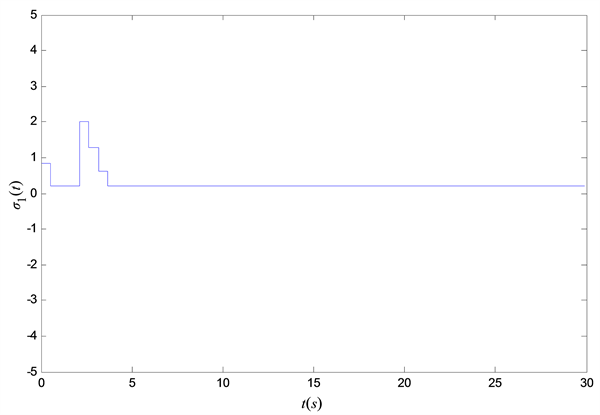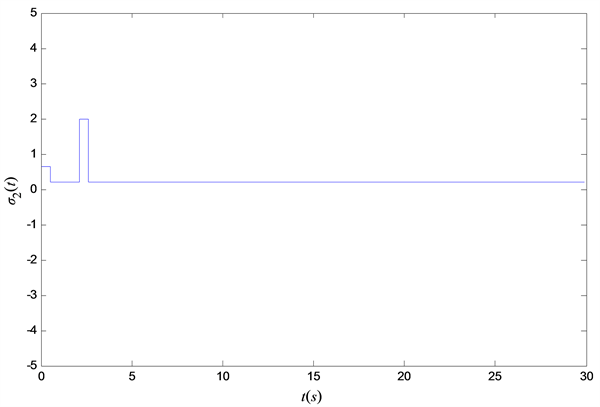Figure 1. The trajectories of the nonlinear function ${\sigma }_{1}\left(t\right),{\sigma }_{2}\left( t \right)$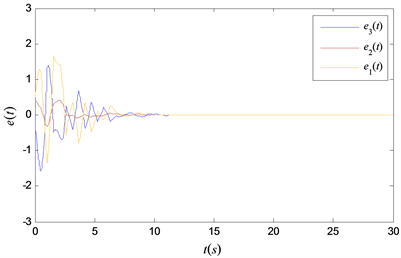Figure 2. Response of e(t) with the sampling period of h = 0.5248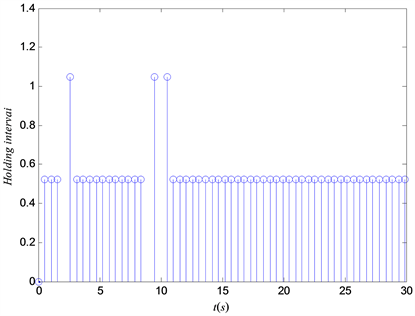Figure 3. Transmission instants and releasing intervals of event-triggered

$\stackrel{˙}{e}\left(t\right)=\left(A+\Delta A\left(t\right)\right)e\left(t\right)+\left(B+\Delta B\left(t\right)\right)e\left(t-\tau \left(t\right)\right)+E\eta \left(De\left(t\right)\right)-KCe\left(t-h\left(t\right)\right),$

$A=\left[\begin{array}{ccc}-7.2& 1.6& 0.8\\ 1& -5.8& 0\\ 3& 11.25& -4\end{array}\right],\text{\hspace{0.17em}}\text{\hspace{0.17em}}\text{\hspace{0.17em}}\text{\hspace{0.17em}}\text{\hspace{0.17em}}\text{\hspace{0.17em}}B=0,\text{\hspace{0.17em}}\text{\hspace{0.17em}}\text{\hspace{0.17em}}\text{\hspace{0.17em}}\text{\hspace{0.17em}}\text{\hspace{0.17em}}E=\left[\begin{array}{c}3.7\\ 5.6\\ 4.1\end{array}\right],\text{\hspace{0.17em}}\text{\hspace{0.17em}}\text{\hspace{0.17em}}\text{\hspace{0.17em}}\text{\hspace{0.17em}}\text{\hspace{0.17em}}C=D={\left[\begin{array}{c}1\\ 0\\ 0\end{array}\right]}^{T},$

${N}_{1}=\left[\begin{array}{ccc}0.1& 0& 0\\ 0& 0.1& 0\\ 0& 0& 0.1\end{array}\right],\text{\hspace{0.17em}}\text{\hspace{0.17em}}\text{\hspace{0.17em}}\text{\hspace{0.17em}}\text{\hspace{0.17em}}\text{\hspace{0.17em}}\text{\hspace{0.17em}}\text{\hspace{0.17em}}\text{\hspace{0.17em}}{N}_{2}=0,\text{\hspace{0.17em}}\text{\hspace{0.17em}}\text{\hspace{0.17em}}\text{\hspace{0.17em}}{N}_{3}=\left[\begin{array}{c}0.1\\ 0\\ 0\end{array}\right],\text{\hspace{0.17em}}\text{\hspace{0.17em}}\text{\hspace{0.17em}}\text{\hspace{0.17em}}\text{\hspace{0.17em}}M=\left[\begin{array}{ccc}1& 0& 0\\ 0& 1& 0\\ 0& 0& 1\end{array}\right]\text{\hspace{0.17em}}.$Table 2. The maximum sampling period h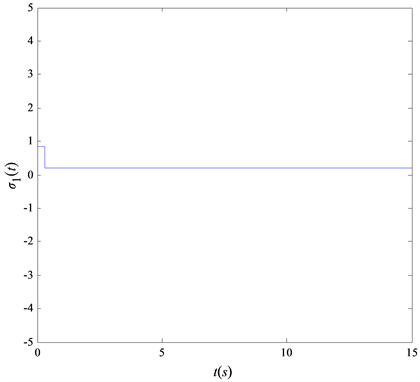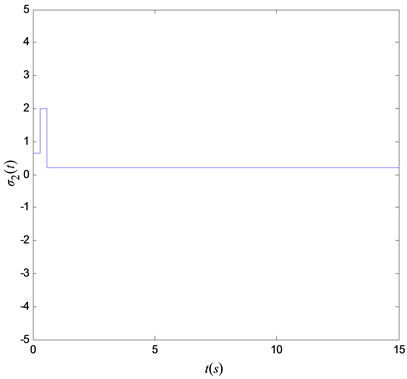Figure 4. The trajectories of the nonlinear function ${\sigma }_{1}\left(t\right),{\sigma }_{2}\left( t \right)$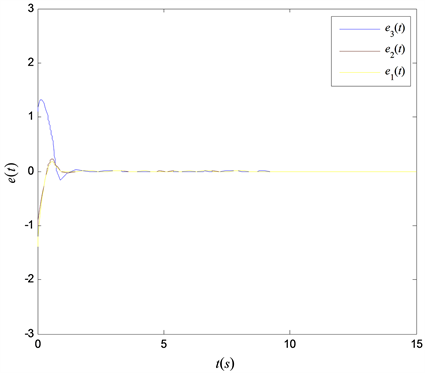Figure 5. Response of e(t) with the sampling period of h = 0.3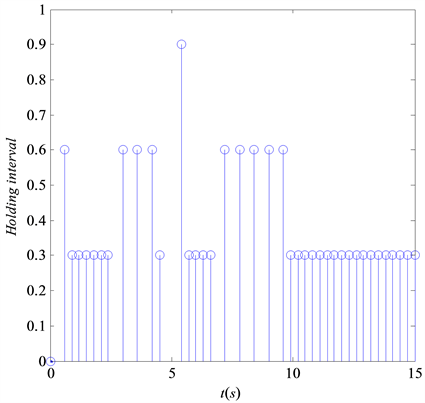Figure 6. Transmission instants and releasing intervals of event-triggered

5. 结论

NOTES

*通讯作者。

  Chen, X., Guan, S., He, Y., et al. (2017) Improved Synchronization of Chaotic Lur’e Systems with Time Delay Using Sampled-Data Control. Journal of the Franklin Institute, 354, 1618-1636. https://doi.org/10.1016/j.jfranklin.2016.12.006  Zhang, R.M., Zeng, D.Q. and Zhong, S.M. (2017) Novel Master-Slave Synchronization Criteria of Chaotic Lur’e Systems with Time Delays Using Sampled-Data Control. Journal of the Franklin Institute, 354, 4930-4954. https://doi.org/10.1016/j.jfranklin.2017.05.008  Park, J. and Park, P. (2018) H∞ Sampled-State Feedback Control for Synchronization of Chaotic Lur’e Systems with Time Delays. Journal of the Franklin Institute, 355, 8005-8026. https://doi.org/10.1016/j.jfranklin.2018.08.019  Zhang, R.M., Zeng, D.Q., Liu, X.Z., et al. (2019) A New Method for Quantized Sampled-Data Synchronization of Delayed Chaotic Lur’e Systems. Applied Mathematical Modelling, 70, 471-489. https://doi.org/10.1016/j.apm.2019.01.041  Shi, K.B., Tang, Y.Y., Liu, X.Z., et al. (2017) Non-Fragile Sampled-Data Robust Synchronization of Uncertain Delayed Chaotic Lurie Systems with Randomly Occurring Controller Gain Fluctuation. ISA Transactions, 66, 185-199. https://doi.org/10.1016/j.isatra.2016.11.002  Shi, K.B., Liu, X.Z., Zhu, H., et al. (2016) Novel Integral Inequality Approach on Master-Slave Synchronization of Chaotic Delayed Lur’e Systems with Sampled-Data Feedback Control. Nonlinear Dynamics, 83, 1259-1274. https://doi.org/10.1007/s11071-015-2401-x  Fridman, E., Shaked, U. and Liu, K. (2009) New Conditions for Delay-Derivative-Dependent Stability. Automatica, 45, 2723-2727. https://doi.org/10.1016/j.automatica.2009.08.002  严欢, 高岩波. 时滞Lur’e系统的采样数据主从同步[J]. 系统科学与数学, 2018, 38(3): 285-307.  Kim, J.-H. (2011) Note on Stability of Linear Systems with Time-Varying Delay. Automatic, 47, 2118-2121. https://doi.org/10.1016/j.automatica.2011.05.023  Song, M.K., Park, J.B. and Joo, Y.H. (2015) Robust Stabilization for Uncertain Markovian Jump Fuzzy Systems Based on Free Weighting Matrix Method. Fuzzy Sets and Systems, 277, 81-96. https://doi.org/10.1016/j.fss.2015.02.004  Selivanov, A. and Fridman, E. (2018) Improved Sampled-Data Implementation of Derivative-Dependent Control. IFAC PapersOnLine, 51, 212-215. https://doi.org/10.1016/j.ifacol.2018.11.107  Jiao, J.M. and Zhang, R. (2020) An Extended Reciprocally Convex Matrix Inequality and Its Application to Stability Analysis of Systems with Additive Time Varying Delays. Journal of the Franklin Institute, 357, 2282-2294. https://doi.org/10.1016/j.jfranklin.2019.11.065  Park, P., Lee, W.I. and Lee, S.Y. (2015) Auxiliary Function-Based Integral Inequalities for Quadratic Functions and Their Applications to Time-Delay Systems. Journal of the Franklin Institute, 352, 1378-1396. https://doi.org/10.1016/j.jfranklin.2015.01.004  Zhang, C.K., He, Y., Jiang, L., et al. (2017) An Extended Reciprocally Convex Matrix Inequality for Stability Analysis of Systems with Time-Varying Delay. Automatica, 85, 481-485. https://doi.org/10.1016/j.automatica.2017.07.056  Liu, S.J. and Zhou, L. (2016) Network Synchronization and Application of Chaotic Lur’e Systems Based on Event-Triggered Mechanism. Nonlinear Dynamics, 83, 2497-2507. https://doi.org/10.1007/s11071-015-2498-y  Shi, P., Wang, H.J. and Lim, C.-C. (2016) Network-Based Event-Triggered Control for Singular Systems with Quantizations. IEEE Transactions on Industrial Electronics, 63, 1230-1238. https://doi.org/10.1109/TIE.2015.2475515  Gu, Z., Shi, P., Yue, D., et al. (2019) Decentralized Adaptive Event-Triggered H-Infinity Filtering for a Class of Networked Nonlinear Interconnected Systems. IEEE Transactions on Cybernetics, 49, 1570-1579. https://doi.org/10.1109/TCYB.2018.2802044  Wang, Y.M., Xiong, L.L., Liu, X.Z., et al. (2017) New Delay-Dependent Synchronization Criteria for Uncertain Lur’e Systems via Time-Varying Delayed Feedback Control. Journal of Nonlinear Sciences and Applications, 354, 1927-1940. https://doi.org/10.22436/jnsa.010.04.52  Zhang, H.M., Cao, J.D. and Xiong, L.L. (2019) Novel Synchronization Conditions for Time-Varying Delayed Lur’e System with Parametric Uncertainty. Applied Mathematics and Computation, 350, 224-236. https://doi.org/10.1016/j.amc.2018.12.073  Li, T., Yuan, R.T., Fei, S.M., et al. (2018) Sampled-Data Synchronization of Chaotic Lur’e Systems via an Adaptive Event-Triggered Approach. Information Sciences, 462, 40-54. https://doi.org/10.1016/j.ins.2018.06.012  EI, G.L., Feron, E. and Balakrishnan, V. (1994) Linear Matrix Inequalities in System and Control Theory. Society for Industrial and Applied Mathematics, Philadelphia.  Tian, E., Yue, D. and Zhang, Y. (2009) Delay-Dependent Robust H∞ Control for T-S Fuzzy System with Interval Time-Varying Delay. Fuzzy Sets and Systems, 160, 1708-1719. https://doi.org/10.1016/j.fss.2008.10.014